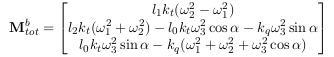# SIH with custom airframe

Hi px4 community, I am trying to test out a custom tricopter airframe. I would like to make use of the SIH Module so I’m trying to set it up for the default tricopter as a start.

I am trying to modify the src/lib/modules/sih.cpp file. There is a section which generates the torques and thrusts, and I have confirmed from @Romain_Chiappinelli that this is the section I need to modify.

Also, I have created a new airframe config for the sih which points to the default tri mixer, rather than the quad_x one. I simply duplicated the config file here PX4-Autopilot/1100_rc_quad_x_sih.hil at master · PX4/PX4-Autopilot · GitHub

Now my question is: for a tricopter, with 3 rotors and 1 servo, looking at the sih.cpp file, I suppose u1,u2,u3 would refer to the motors, and u4 would refer to the tail servo?

Thank you

Hi Daniel,

You can set your custom forces in Sih::generate_force_and_torques().

As for the mixer tri_y_yaw+, yes the motors are u1 u2 u3 and the servo is u4.

Be careful, u4 should be in the range [-1;1], you may want to modify Sih::read_motors() to include something like this.

Best,

Romain

HI @Romain_Chiappinelli , thanks once a gain for your response. Firstly, I’m a total beginner with c++. However, I can program in matlab and a bit in python so I have tried to joggle a few things as follows:

• I added a new parameter SIH_L_ARM in the src/modules/sih/sih_params.c file, this is because the tricopter has three different lengths, one corresponding to the roll, two separate ones which relate to pitch, where I have assumed the one between the two front rotors and CG along x-direction to be L_pitch and then the new length I defined is the one between the tail rotor and the CG along the x-direction, which is the length of each arm in essence.
• Added new default values for L_ROLL and L_PITCH.
• Modified the sih_parameters_updated() function to include the newly added parameter.
• Changed the model to that representing a tricopter

A snap shot of all these is below:

void Sih::parameters_updated()
{
_T_MAX = _sih_t_max.get();
_Q_MAX = _sih_q_max.get();
_L_ROLL = _sih_l_roll.get();
_L_PITCH = _sih_l_pitch.get();
_L_ARM = _sih_l_arm.get();
_KDV = _sih_kdv.get();
_KDW = _sih_kdw.get();
_H0 = _sih_h0.get();

{
actuator_outputs_s actuators_out;

``````if (_actuator_out_sub.update(&actuators_out)) {
for (int i = 0; i < NB_MOTORS; i++) { // saturate the motor signals
if (i < NB_MOTORS) {

float u_sp = constrain((actuators_out.output[i] - PWM_DEFAULT_MIN) / (PWM_DEFAULT_MAX - PWM_DEFAULT_MIN), 0.0f, 1.0f);

} else {

float u_sp = constrain((actuators_out.output[i] - PWM_DEFAULT_MIN) / ((PWM_DEFAULT_MAX - PWM_DEFAULT_MIN), -1.0f, 1.0f);
}

_u[i] = _u[i] + _dt / _T_TAU * (u_sp - _u[i]); // first order transfer function with time constant tau
}
}
``````

}

Please see if the read_motors() one makes sense as per limiting the servo to {-1,1}.

void Sih::generate_force_and_torques()
{
_T_B = Vector3f(0.0f, 0.0f, -_T_MAX * (+_u + _u + _u * cos(_u)));
_Mt_B = Vector3f(_L_ROLL * _T_MAX * (-_u + _u),
_L_PITCH * _T_MAX * (+_u + _u) - (_L_ARM * _T_MAX * (_u * cos(_u))) - (_Q_MAX * (_u * sin(_u))),
_Q_MAX * (-_u - _u - _u * cos(_u)) + (_L_ARM * _T_MAX * (_u * sin(_u)));

The corresponding equations are:So Ti = kt x omega_i^2, and Qi = kq x omega_i^2, where i = 1,2,3. Note I ignored Fy.

You can view it better on my fork here: PX4-Autopilot/sih.cpp at sih_tri · DanAbara/PX4-Autopilot · GitHub